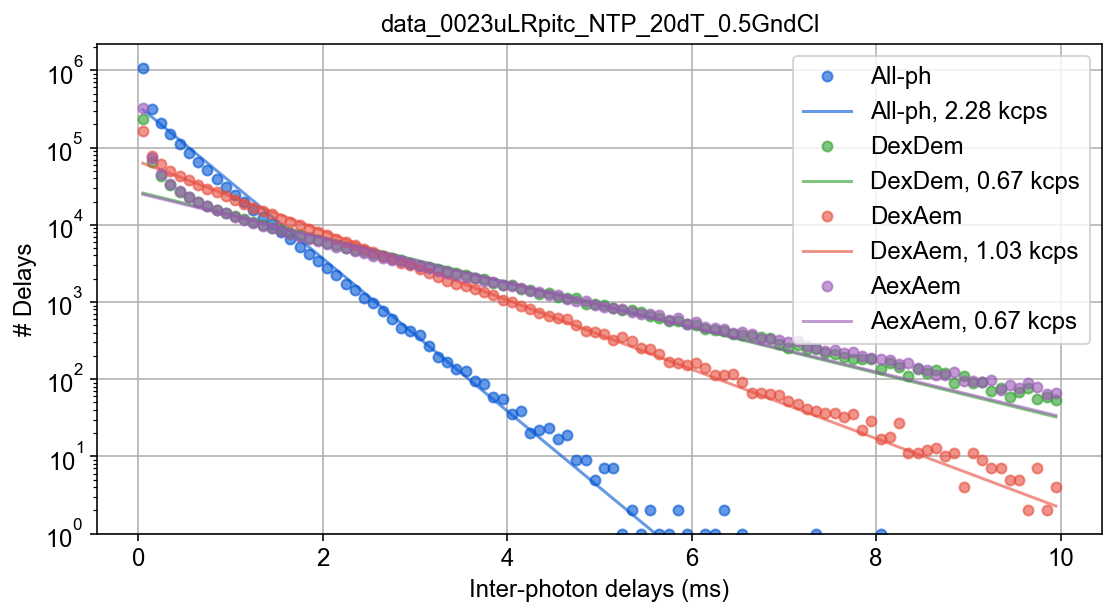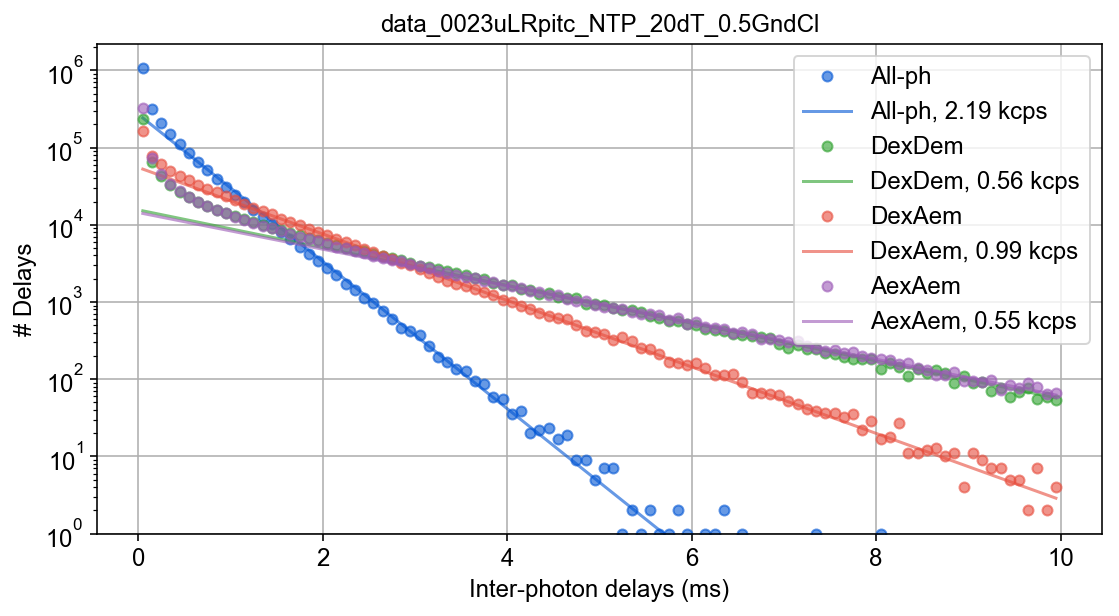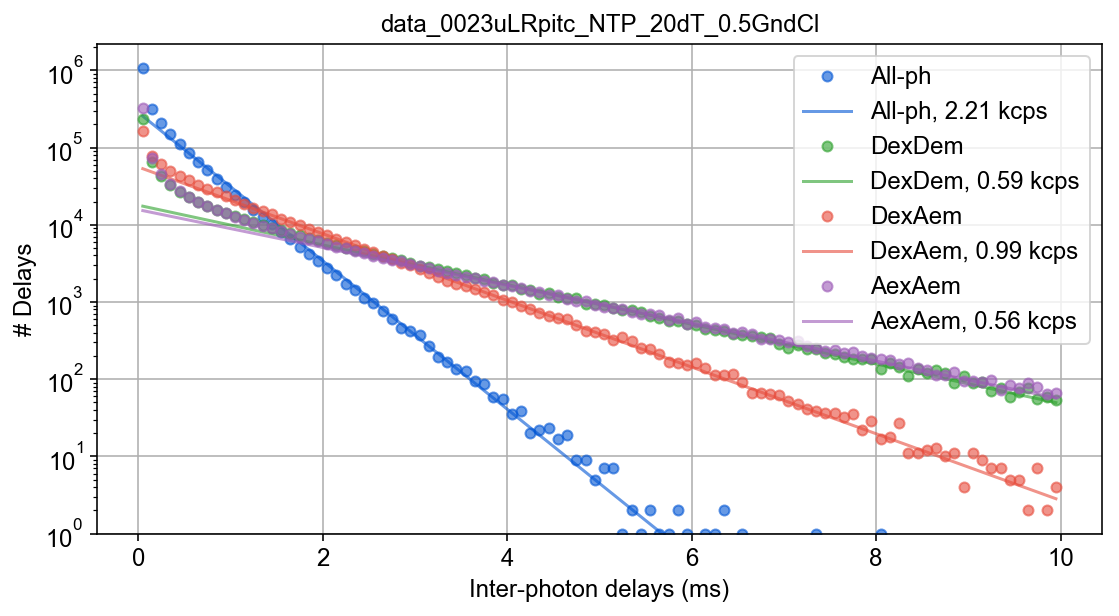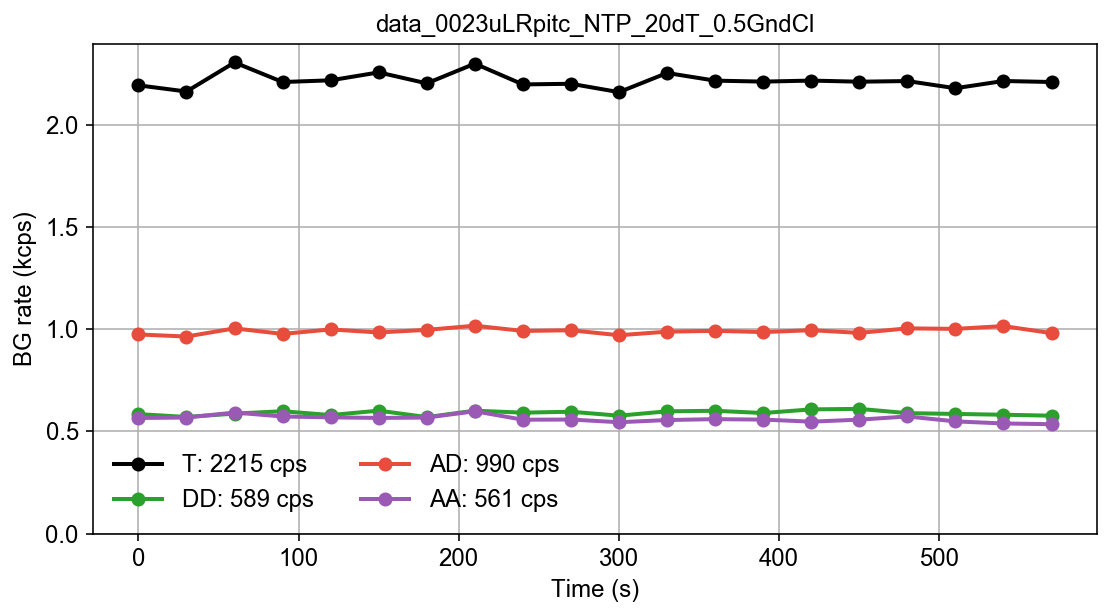# Example - Background estimation¶

This notebook is part of smFRET burst analysis software FRETBursts.

In this notebook, we show different ways of computing, plotting and exporting background data. For a complete tutorial on burst analysis see FRETBursts - us-ALEX smFRET burst analysis.

In :
import pandas as pd
from fretbursts import *

 - Optimized (cython) burst search loaded.
- Optimized (cython) photon counting loaded.
--------------------------------------------------------------
You are running FRETBursts (version 0.6.5).

If you use this software please cite the following paper:

FRETBursts: An Open Source Toolkit for Analysis of Freely-Diffusing Single-Molecule FRET
Ingargiola et al. (2016). http://dx.doi.org/10.1371/journal.pone.0160716

--------------------------------------------------------------

In :
sns = init_notebook(apionly=True)
print('seaborn version: ', sns.__version__)

seaborn version:  0.8.0

In :
# Tweak here matplotlib style
import matplotlib as mpl
mpl.rcParams['font.sans-serif'].insert(0, 'Arial')
mpl.rcParams['font.size'] = 12
%config InlineBackend.figure_format = 'retina'


# Retrieve the data¶

In :
url = 'http://files.figshare.com/2182601/0023uLRpitc_NTP_20dT_0.5GndCl.hdf5'
full_fname = "./data/0023uLRpitc_NTP_20dT_0.5GndCl.hdf5"


URL:  http://files.figshare.com/2182601/0023uLRpitc_NTP_20dT_0.5GndCl.hdf5
File: 0023uLRpitc_NTP_20dT_0.5GndCl.hdf5

# Total photons (after ALEX selection):     2,259,522
#  D  photons in D+A excitation periods:      721,537
#  A  photons in D+A excitation periods:    1,537,985
# D+A photons in  D  excitation period:     1,434,842
# D+A photons in  A  excitation period:       824,680



# Background estimation¶

The first step of the analysis is estimating the background. The assumption is that the background is a Poisson process and therefore the corresponding inter photon delays are exponentially distributed. Since the background can change during the measurement, a new estimation is computed every time_s seconds (this time is called the background period).

The inter-photon delay distribution contains both single-molecule signal and background. The single-molecule signal produces a inter-photon delays at short time scales, while the background produces an exponential tail extenting to longer timescales. Hence, we need a threshold to discriminate between the exponential tail and the single-molecule peak.

FRETBursts provides several ways to specify the minimum threshold and different functions to fit the exponential tail.

The reference documentation is the following:

## Single threshold¶

Let start with a standard Maximum Likelihood (ML) background fit with a minimum tail threshold of 500 μs:

In :
d.calc_bg(bg.exp_fit, time_s=1000, tail_min_us=500)

 - Calculating BG rates ... [DONE]


We can look at how the fit looks with:

In :
dplot(d, hist_bg, show_fit=True);Note that the fits are not very good. This is understandable because we used a single threshold for all the photon streams, each one having a quite different background.

## Multiple thresholds¶

To improve the fit, we can try specifying a threshold for each channel. This method is bit ad-hoc but it may work well when the thresholds are properly choosen.

In :
d.calc_bg(bg.exp_fit, time_s=1000, tail_min_us=(800, 4000, 1500, 1000, 3000))

 - Calculating BG rates ... [DONE]

In :
dplot(d, hist_bg, show_fit=True);For ALEX measurements, the tuple passed to tail_min_us in order to define the thresholds needs to contain
5 values corresponding the 5 distinct photon streams (the all-photon stream plus the 4 base alternation streams). The order of these 5 values need to match the order of photon streams in the Data.ph_streams attribute:

In :
d.ph_streams

Out:
[Ph_sel(Dex='DAem', Aex='DAem'),
Ph_sel(Dex='Dem', Aex=None),
Ph_sel(Dex='Aem', Aex=None),
Ph_sel(Dex=None, Aex='Dem'),
Ph_sel(Dex=None, Aex='Aem')]

## Automatic threshold¶

Finally, is possible to let FRETBursts infer the threshold automatically (recommended) with:

In :
d.calc_bg(bg.exp_fit, time_s=1000, tail_min_us='auto', F_bg=1.7)

 - Calculating BG rates ... [DONE]


Which results in the following fit plot:

In :
dplot(d, hist_bg, show_fit=True);Under the hood, this method estimates the threshold according to this formula:

threshold_auto = F_bg / coarse_background_rate



where F_bg is an input argument (by default 1.7) and coarse_background_rate is an initial background estimation performed with a fixed threshold. This method is concemptually an iterative method to compute the threshold that is stopped after the second iteration (this is usually more than enough for accurate estimates).

This latter method is the recommended, since it works well and without user intervention in a wide range of experimental conditions.

## Background timetrace¶

It is a good practice to monitor background rates as a function of time. Here, we compute background in adjacent 30s windows (called background periods) and plot the estimated rates as a function of time.

In :
d.calc_bg(bg.exp_fit, time_s=30, tail_min_us='auto', F_bg=1.7)

 - Calculating BG rates ... [DONE]

In :
dplot(d, timetrace_bg);## Getting the background rates¶

The background rates are stored in Data() attribute bg, a dict with photon streams (Ph_sel objects) as key. Each item in the dict contains a list of fitted background rates for each channel and period.

In :
d.bg

Out:
{Ph_sel(Dex='DAem', Aex='DAem'): [array([ 2192.33771456,  2162.14083635,  2304.31199333,  2208.57734993,
2216.88852285,  2255.68759074,  2202.02910176,  2298.45997825,
2196.7975027 ,  2199.98590374,  2159.05120194,  2252.82753492,
2215.34278931,  2210.60163812,  2215.6184806 ,  2210.1510978 ,
2213.32385234,  2178.18199206,  2213.60242855,  2208.64479515])],
Ph_sel(Dex='Dem', Aex=None): [array([ 584.18970691,  570.58641253,  586.80564353,  598.45561342,
579.68811571,  601.34029113,  569.74563784,  601.248969  ,
591.47186394,  596.06342699,  576.64661876,  598.0513001 ,
600.65952237,  589.70963389,  607.49394193,  610.00901454,
589.13559128,  585.2150928 ,  581.32356205,  576.791585  ])],
Ph_sel(Dex='Aem', Aex=None): [array([  973.96356856,   963.80151895,  1003.656506  ,   976.56430321,
998.56747257,   983.73415654,   996.47590969,  1016.73812567,
991.14920045,   994.47939727,   970.14966698,   987.63026179,
991.74747802,   985.56352863,   994.77741329,   981.68939719,
1003.74305566,  1001.2290634 ,  1015.02463123,   980.3738204 ])],
Ph_sel(Dex=None, Aex='Dem'): [array([ 81.25392506,  77.34935176,  70.85741407,  73.75133485,
76.24222792,  77.82545767,  73.1705312 ,  78.46092124,
70.92503618,  71.6808552 ,  75.55277658,  77.84662415,
74.29129798,  69.49896567,  74.36290656,  77.00695872,
74.03801857,  72.81002019,  73.92609799,  74.57414299])],
Ph_sel(Dex=None, Aex='Aem'): [array([ 565.41275495,  567.69856299,  592.02680332,  572.78126164,
568.49858848,  565.73474853,  567.51131955,  598.30138811,
556.9935006 ,  557.87730382,  544.68106085,  555.38189993,
559.75536058,  557.55247497,  547.56454569,  557.25229028,
572.92889217,  548.83714375,  538.59524696,  535.0871576 ])]}

We can also get the average background for each channel:

In :
d.bg_mean

Out:
{Ph_sel(Dex='DAem', Aex='DAem'): [2215.7281152497922],
Ph_sel(Dex='Dem', Aex=None): [589.73157718553762],
Ph_sel(Dex='Aem', Aex=None): [990.55292377373189],
Ph_sel(Dex=None, Aex='Dem'): [74.771243227050803],
Ph_sel(Dex=None, Aex='Aem'): [561.52361523921024]}
In :
bg_data = pd.DataFrame({str(k): v for k, v in d.bg.items()})
bg_data

Out:
AexAem AexDem DexAem DexDem all
0 565.412755 81.253925 973.963569 584.189707 2192.337715
1 567.698563 77.349352 963.801519 570.586413 2162.140836
2 592.026803 70.857414 1003.656506 586.805644 2304.311993
3 572.781262 73.751335 976.564303 598.455613 2208.577350
4 568.498588 76.242228 998.567473 579.688116 2216.888523
... ... ... ... ... ...
15 557.252290 77.006959 981.689397 610.009015 2210.151098
16 572.928892 74.038019 1003.743056 589.135591 2213.323852
17 548.837144 72.810020 1001.229063 585.215093 2178.181992
18 538.595247 73.926098 1015.024631 581.323562 2213.602429
19 535.087158 74.574143 980.373820 576.791585 2208.644795

20 rows × 5 columns

# Burst analysis¶

After background estimation, you are ready to perform burts search.

If you want more details see Burst analysis.

Executed: Tue Nov 21 22:58:18 2017

Duration: 8 seconds.

Autogenerated from: Example - Background estimation.ipynb